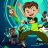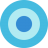# The Range Indicator by Jack Weinberg for ThinkorSwim#### BenTen

Staff
VIP
Developed by Jack Weinberg, Range indicator compares intraday range with inter-day range. Author had a strong belief that crossing of intraday range outside the inter-day range is an indication of end of current trend

Interpretation
• RI crossing above level 80 is a signal to exit
• RI below 20 is indication that a new trend is about to take charge
• RI is useful to filter signal given by other studies
It oscillates between 0 to 100 levels### thinkScript Code

Code:
``````# The Range Indicator by Jack Weinberg
# Assembled by BenTen at useThinkScript.com

declare lower;

input stochper = 10;
input smoothper = 3;
input highline = 80;
input lowline = 20;

def a = atr(1);
def Value1 = if(close > close, a / ((close - close) + 0.00001), a);
def Value2 = lowest(Value1, stochper);
def Value3 = highest(Value1, stochper);
def StochRange = if((Value3 - Value2) > 0, 100 * (Value1 - Value2) / ((Value3 - Value2)  + 0.00001), 100 * (Value1 - Value2));

def TRI = expAverage(StochRange, smoothper);

plot line = TRI;
plot hl = highline;
plot ll = lowline;``````The Logical Trader's Pivot Range Indicator for ThinkorSwim Custom 8Custom indicator in watchlist opening range Questions 4